Courses

# NCERT Solutions - Basic Concepts of Chemistry (Part - 1) Class 11 Notes | EduRev

## Class 11: NCERT Solutions - Basic Concepts of Chemistry (Part - 1) Class 11 Notes | EduRev

The document NCERT Solutions - Basic Concepts of Chemistry (Part - 1) Class 11 Notes | EduRev is a part of the Class 11 Course NCERTs for NEET.
All you need of Class 11 at this link: Class 11

Question 1.1: Calculate the molecular mass of the following:
(a) H2O
(b) CO2
(c) CH4

(a) H2O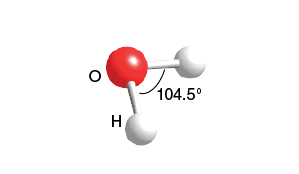H2O StructureThe molecular mass of water, H2O:
= (2 × Atomic mass of hydrogen) + (1 × Atomic mass of oxygen)
= [2(1.0084)  + 1(16.00 u)]
= 2.016 u + 16.00 u
= 18.016
= 18.02 u
(b) CO2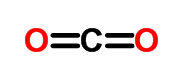COStructure

The molecular mass of carbon dioxide, CO2:

= (1 × Atomic mass of carbon) + (2 × Atomic mass of oxygen)

= [1(12.011 u) + 2 (16.00 u)]

= 12.011 u +  32.00 u

= 44.01 u

(c) CH4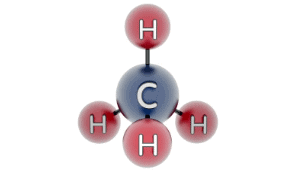CHStructure

The molecular mass of methane, CH4:

= (1 × Atomic mass of carbon) + (4 × Atomic mass of hydrogen)

= [1(12.011 u) + 4 (1.008 u)]

= 12.011 u + 4.032 u

= 16.043 u

Question 1.2: Calculate the mass per cent of different elements present in sodium sulfate (Na2SO4).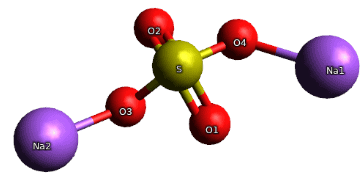Na2SO4

The molecular formula of sodium sulphate is Na2SO4
Molar mass of Na2SO4 = [(2 × 23.0) + (32.066) + 4 (16.00)]
= 142.066 g

Mass percent of an element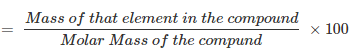∴ Mass percent of sodium: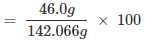= 32.379
= 32.4%
Mass percent of sulphur: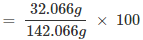= 22.57
= 22.6 %
Mass percent of oxygen: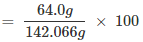= 45.049
= 45.05%

Question 1.3: Determine the empirical formula of an oxide of iron that has 69.9% iron & 30.1% dioxygen by mass.

Given:
% of iron by mass = 69.9 %
% of oxygen by mass = 30.1 %
Relative moles of iron in iron oxide: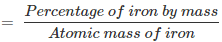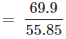= 1.25
Relative moles of oxygen in iron oxide: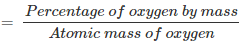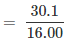= 1.88
The simplest molar ratio of iron to oxygen:
= 1.25: 1.88
= 1:1.5 = 2:3
∴ The empirical formula of the iron oxide is Fe2O3.

Question 1.4: Calculate the amount of carbon dioxide that could be produced when
(a) 1 mole of carbon is burnt in the air.
(b) 1 mole of carbon is burnt in 16 g of dioxygen.
(c) 2 moles of carbon are burnt in 16 g of dioxygen.

The balanced reaction of combustion of carbon can be written as: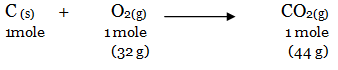(a) As per the balanced equation, 1 mole of carbon burns in 1 mole of dioxygen (air) to produce 1 mole of carbon dioxide.
(b) According to the question, only 16 g (0.5 mol) of dioxygen is available. Hence, it will react with 0.5 moles of carbon to give 22 g of carbon dioxide. Hence, it is a limiting reactant.
(c) Since oxygen is the limiting reactant here, the 16g (0.5 mol) of O2 will react with 6 g of carbon (0.5 mol) to form 22 g of carbon dioxide. The remaining 18 g of carbon (1.5 mol) will not undergo combustion.

Question 1.5: Calculate the mass of sodium acetate (CH3COONa) required to make 500 mL of 0.375 molars aqueous solution. The molar mass of sodium acetate is 82.0245 g mol–1.

0.375 M aqueous solution of sodium acetate ≡ 1000 mL of a solution containing 0.375 moles of sodium acetate.
∴ A number of moles of sodium acetate in 500 mL.
= 0.375/1000 x 500
= 0.1875 mole
Molar mass of sodium acetate = 82.0245 g mol–1 (Given)
∴ Required mass of sodium acetate = (82.0245 g mol–1) (0.1875 mole)
= 15.38 g

Question 1.6: Calculate the concentration of nitric acid in moles per litre in a sample that has a density, 1.41 g mL–1, and the mass percent of nitric acid in it being 69%.

The mass percent of nitric acid in the sample = 69 % (Given)
Thus, 100 g of nitric acid contains 69 g of nitric acid by mass.
Molar mass of nitric acid (HNO3):
= [1 +14 + 3(16)] g mol–1
= 1 +14 + 48
= 63 g mol–1
∴ Number of moles in 69 g of HNO3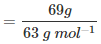= 1.095 mol
The volume of 100 g of nitric acid solution: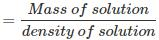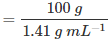= 70.92 mL ≡ 70.92 x 10-3 L
The concentration of nitric acid: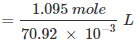= 15.44 mol/L
∴ Concentration of nitric acid = 15.44 mol/L

Question 1.7: How much copper can be obtained from 100 g of copper sulphate (CuSO4)?

1 mole of CuSO4 contains 1 mole of copper.
Molar mass of CuSO4 = (63.5) + (32.00) + 4(16.00)
= 63.5 +32.00 + 64.00
= 159.5 g
159.5 g of CuSO4 contains 63.5 g of copper.
⇒ 100 g of CuSO4 will contain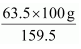of copper.
∴ Amount of copper that can be obtained from 100 g CuSO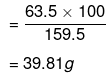Question 1.8: Determine the molecular formula of an oxide of iron in which the mass per cent of iron and oxygen are 69.9 and 30.1, respectively. Given that the molar mass of the oxide is 159.69 g mol–1.

Mass percent of iron (Fe) = 69.9% (Given)
Mass percent of oxygen (O) = 30.1% (Given)
Number of moles of iron present in the oxide = 69.90/55.85 = 1.25
Number of moles of oxygen present in the oxide = 30.1/16.0 = 1.88
The ratio of iron to oxygen in the oxide: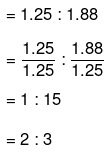The empirical formula of the oxide is Fe2O3.
Empirical formula mass of Fe2O3 = [2(55.85) + 3(16.00)] g
Molar mass of Fe2O3 = 159.69 g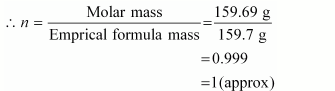The molecular formula of a compound is obtained by multiplying the empirical formula with n.
Thus, the empirical formula of the given oxide is Fe2O3 and n is 1.
Hence, the molecular formula of the oxide is Fe2O3.

Question 1.9: Calculate the atomic mass (average) of chlorine using the
following data: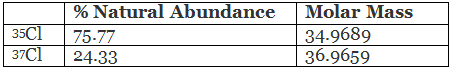The average atomic mass of chlorine: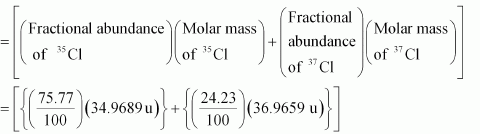= 26.4959 + 8.9568

= 35.4527 u

The average atomic mass of chlorine = 35.4527 u

Question 1.10: In three moles of ethane (C2H6), calculate the following:

(a) Number of moles of carbon atoms.

(b) Number of moles of hydrogen atoms.

(c) Number of molecules of ethane.

(a) 1 mole of C2H6 contains 2 moles of carbon atoms.

Number of moles of carbon atoms in 3 moles of C2H

= 2 × 3 = 6

(b) 1 mole of C2Hcontains 6 moles of hydrogen atoms.

Number of moles of carbon atoms in 3 moles of C2H

= 3 × 6 = 18

(c) 1 mole of C2Hcontains 6.023 × 1023 molecules of ethane.

Number of molecules in 3 moles of C2H

= 3 × 6.023 × 1023

= 18.069 × 102

Question 1.11: What is the concentration of sugar (C12H22O11) in mol L–1 if its 20 g are dissolved in enough water to make a final volume up to 2 L?

Molarity (M) of a solution is given by: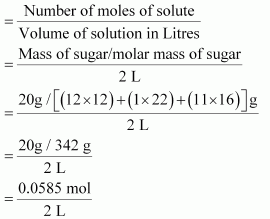= 0.02925 mol L–1

The molar concentration of sugar = 0.02925 mol L–1

Question 1.12: If the density of methanol is 0.793 kg L–1, what is its volume needed for making 2.5 L of its 0.25 M solution?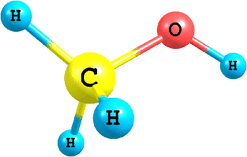CH3OH Molar mass of methanol (CH3OH) = (1 × 12) + (4 × 1) + (1 × 16)

= 32 g mol–1

= 0.032 kg mol–1

Molarity of methanol solution: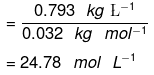(Since density is mass per unit volume)

Applying,

M1V1 = M2V2
(Given solution) = (Solution to be prepared)

(24.78 mol L–1) V= (2.5 L) (0.25 mol L–1)

V= 0.0252 L

V= 25.22 mL

Question 1.13: The pressure is determined as force per unit area of the surface.

an SI unit of pressure, Pascal is as shown below:

• 1 Pa = 1 N m–2

If the mass of air at sea level is 1034 g cm–2, calculate the pressure in Pascals.

The pressure is defined as a force acting per unit area of the surface.

P = F/A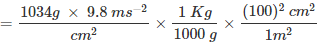= 1.01332 × 105 kg m-1 s-2

We know,

1 N = 1 kg ms–2

Then,

1 Pa = 1 N m–2 = 1 kg m–2s–2

1 Pa = 1 kg m1s2

∴ Pressure = 1.01332 × 105 Pa.

Question 1.14: What is the SI unit of mass? How is it defined?

The SI unit of mass is the kilogram (kg)

• 1 Kilogram is defined as the mass equal to the mass of the international prototype of the kilogram.

Question 1.15: Match the following prefixes with their multiples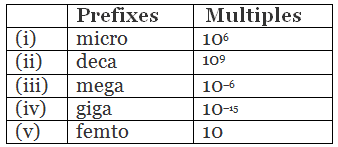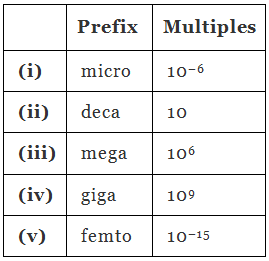Question 1.16: What do you mean by significant figures?

Significant figures are those meaningful digits that are known with certainty. They indicate uncertainty in an experiment or calculated value.
Example: If 15.6 mL is the result of an experiment, then 15 is certain while 6 is uncertain, and the total number of significant figures is 3.
Hence, significant figures are defined as the total number of digits in a number, including the last digit that represents the uncertainty of the result.

Question 1.17: A sample of drinking water was found to be severely contaminated with chloroform, CHCl3, supposed to be carcinogenic in nature. The level of contamination was 15 ppm (by mass).
(a) Express this in per cent by mass.
(b) Determine the molality of chloroform in the water sample.

(a) 1 ppm is equivalent to 1 part out of 1 million (106) parts.
The mass percent of 15 ppm chloroform in water:
= 15/106 × 100
≈1.5 x 10-3%
(b) 100 g of the sample contains 1.5 × 10–3 g of CHCl3.
⇒ 1000 g of the sample contains 1.5 × 10–2 g of CHCl3.
The molality of chloroform in water: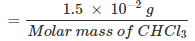Molar mass of CHCl3 = 12.00 + 1.00 + 3(35.5)
= 119.5 g mol–1
Molality of chloroform in water = 0.0125 × 10–2 m
= 1.25 × 10–4 m

Question 1.18: Express the following in the scientific notation:
(a) 0.0048
(b) 234,000
(c) 8008
(d) 500.0
(e) 6.0012

(a) 0.0048 = 4.8× 10–3
(b) 234, 000 = 2.34 ×105
(c) 8008 = 8.008 ×103
(d) 500.0 = 5.000 × 102
(e) 6.0012 = 6.0012

Question 1.19: How many significant figures are present in the following?
(a) 0.0025
(b) 208
(c) 5005
(d) 126,000
(e) 500.0
(f) 2.0034

(a) 0.0025
There are 2 significant figures.
(b) 208
There are 3 significant figures.
(c) 5005
There are 4 significant figures.
(d) 126,000
There are 3 significant figures.
(e) 500.0
There are 4 significant figures.
(f) 2.0034
There are 5 significant figures.

Question 1.20: Round up the following up to three significant figures:
(a) 34.216
(b) 10.4107
(c) 0.04597
(d) 2808

(a) 34.2
(b) 10.4
(c) 0.0460
(d) 2810

Question 1.21: The following data are obtained when dinitrogen and dioxygen react together to form different compounds: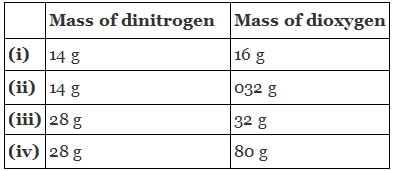(a) Which law of chemical combination is obeyed by the above experimental data? Give its statement.
(b) Fill in the blanks in the following conversions:
(i) 1 km = ........... mm = ........... pm
(ii) 1 mg = ...........  kg = ...........  ng
(iii) 1 ml = ...........  l = ...........  dm3

(a) If we fix the mass of dinitrogen at 28 g, then the masses of dioxygen that will combine with the fixed mass of dinitrogen are 32 g, 64 g, 32 g, and 80 g.
The masses of dioxygen bear a whole number ratio of 1:2:2:5. Hence, the given experimental data obeys the law of multiple proportions.

The law states that if two elements combine to form more than one compound, then the masses of one element that combines with the fixed mass of another element are in the ratio of small whole numbers.

(b) (i) 1 km = 1 km × 1000 m/1 km × 100 cm/1m × 10 mm/1cm
⇒ 1 km = 106 mm
⇒ 1 km = 1 km × 1000 m/1 km × 1 pm/10-12 m
⇒ 1 km = 1015 pm
Hence, 1 km = 106 mm = 1015 pm
(ii) 1 mg = 1 mg × 1 g/1000 mg × 1 kg/1000 g
⇒ 1 mg = 10-6 kg
⇒ 1 mg = 1 mg × 1 g/ 1000 mg × 1 mg/ 10-9 g 1
⇒ 1 mg = 106 ng
Hence, 1 mg = 10–6 kg = 106 ng
(iii) 1 mL = 1 mL × 1 L/1000 mL
⇒ 1 mL = 10–3 L
⇒ 1 mL = 1 cm3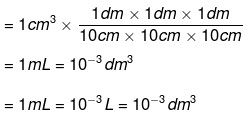Question 1.22: If the speed of light is 3.0 × 108 ms-1, calculate the distance covered by light in 2.00 ns.

According to the question:
Time taken to cover the distance = 2.00 ns = 2.00 × 10–9 s
Speed of light = 3.0 × 108 ms–1
Distance travelled by light in 2.00 ns:
= Speed of light × Time taken
= (3.0 × 108 ms –1) (2.00 × 10–9 s)
= 6.00 × 10–1 m
= 0.600 m

Question 1.23: In a reaction A + B2 → AB2
Identify the limiting reagent, if any, in the following reaction mixtures.
(a) 300 atoms of A + 200 molecules of B2
(b) 2 mol A + 3 mol B2
(c) 100 atoms of A + 100 molecules of B2
(d) 5 mol A + 2.5 mol B
(e) 2.5 mol A + 5 mol B

A limiting reagent determines the extent of a reaction. It is the reactant which is the first to get consumed during a reaction, thereby causing the reaction to stop and limiting the amount of products formed.

(a) According to the given reaction, 1 atom of A reacts with 1 molecule of B. Thus, 200 molecules of B will react with 200 atoms of A, thereby leaving 100 atoms of A unused. Hence, B is the limiting reagent.

(b) According to the reaction, 1 mol of A reacts with 1 mol of B. Thus, 2 mol of A will react with only 2 mol of B. As a result, 1 mol of A will not be consumed. Hence, A is the limiting reagent.

(c) According to the given reaction, 1 atom of A combines with 1 molecule of B. Thus, all 100 atoms of A will combine with all 100 molecules of B. Hence, the mixture is stoichiometric where no limiting reagent is present.

(d) 1 mol of atom A combines with 1 mol of molecule B. Thus, 2.5 mol of B will combine with only 2.5 mol of A. As a result, 2.5 mol of A will be left as such. Hence, B is the limiting reagent.

(e) According to the reaction, 1 mol of atom A combines with 1 mol of molecule B. Thus, 2.5 mol of A will combine with only 2.5 mol of B and the remaining 2.5 mol of B will be left as such. Hence, A is the limiting reagent.

Question 1.24: Dinitrogen and dihydrogen react with each other to produce ammonia according to the following chemical equation:
N2(g) + H2(g) → 2NH3(g)
(a) Calculate the mass of ammonia produced if 2.00 × 103 g dinitrogen reacts with 1.00 × 103 g of dihydrogen.
(b) Will any of the two reactants remain unreacted?
(c) If yes, which one and what would be its mass?

(a) Balancing the given chemical equation,
N2(g) + H2(g) → 2NH3(g)
From the equation, 1 mole (28 g) of dinitrogen reacts with 3 mole (6 g) of dihydrogen to give 2 mole (34 g) of ammonia.
⇒ 2.00 × 103 g of dinitrogen will react with 6 g/28 g × 2.00 x 103 g dihydrogen i.e., 2.00 × 103 g of dinitrogen will react with 428.6 g of dihydrogen.

Given,
Amount of dihydrogen = 1.00 × 103 g

Hence, N2 is the limiting reagent. 28 g of N2 produces 34 g of NH3.
Hence, mass of ammonia produced by:

2000 g of N2 = 34 g/28 g × 2000g
= 2428.57 g

(b) N2 is the limiting reagent and H2 is the excess reagent. Hence, H2 will remain unreacted.

(c) Mass of dihydrogen left unreacted = 1.00 × 103 g – 428.6 g = 571.4 g

Question 1.25: How are 0.50 mol Na2CO3 and 0.50 M Na2CO3 different?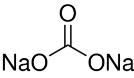Molar mass of Na2CO3 = (2 × 23) +12.00 + (3 × 16) = 106 g mol–1
Now, 1 mole of Na2CO3 means 106 g of Na2CO3.
⇒ 0.5 mol of Na2CO3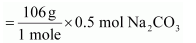= 53 g Na2CO3

⇒ 0.50 M of Na2CO3 = 0.50 mol/L Na2CO3
Hence, 0.50 mol of Na2CO3 is present in 1 L of water or 53 g of Na2CO3 is present in 1 L of water.

Question 1.26: If ten volumes of dihydrogen gas react with five volumes of dioxygen gas, how many volumes of water vapour would be produced?

Reaction of dihydrogen with dioxygen can be written as:
2H2(g) + O2(g) → 2H2O(g)
Now, two volumes of dihydrogen react with one volume of dihydrogen to produce two volumes of water vapour.
Hence, ten volumes of dihydrogen will react with five volumes of dioxygen to produce ten volumes of water vapour.

Question 1.27: Convert the following into basic units:
(a) 28.7 pm
(b) 15.15 pm
(c) 25365 mg

(a) 28.7 pm

1 pm = 10–12 m
28.7 pm = 28.7 × 10–12 m
= 2.87 × 10–11 m

(b) 15.15 pm

1 pm = 10–12 m
15.15 pm = 15.15 × 10–12 m
= 1.515 × 10–11 m

(c) 25365 mg

1 mg = 10–3 g
25365 mg = 2.5365 × 104 × 10–3 g

Since,
1 g = 10–3 kg
2.5365 × 10g = 2.5365 × 10–1 × 10–3 kg
25365 mg = 2.5365 × 10–2 kg.

Question 1.28: Which one of the following will have largest number of atoms?
(a) 1 g Au (s)
(b) 1 g Na (s)
(c) 1 g Li (s)
(d) 1 g of Cl2(g)

(a) 1 g of Au (s)

= 1/197 mol of Au (s) = 6.022 × 1023/197
atoms of Au (s) = 3.06 × 1021 atoms of Au (s)

(b) 1 g of Na (s) = 1/23 mol of Na (s)

= 6.022 × 1023/23  atoms of Na (s)
= 0.262 × 1023 atoms of Na (s)
= 26.2 × 1021 atoms of Na (s)

(c) 1 g of Li (s) = 1/mol of Li (s)

= 6.022 × 1023/7 atoms of Li (s)
= 0.86 × 1023 atoms of Li (s)
= 86.0 × 1021 atoms of Li (s)

(d) 1 g of Cl2 (g) = 1/71  mol of Cl2 (g)
(Molar mass of Cl2 molecule = 35.5 × 2 = 71 g mol–1)

= 6.022 x 1023/71
= 0.0848 × 1023 molecules of Cl2 (g)
= 8.48 × 1021 molecules of Cl2 (g)

As one molecule of Clcontains two atoms of Cl.
Number of atoms of Cl = 2 × 8.48 × 1021 = 16.96 × 1021  atoms of Cl
Hence, 1 g of Li (s) will have the largest number of atoms.

Question 1.29: Calculate the molarity of a solution of ethanol in water in which the mole fraction of ethanol is 0.040 (assume the density of water to be one).

Mole fraction of C2H5OH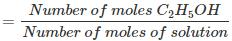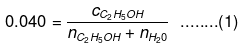Number of moles present in 1 L water: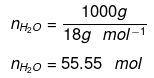Substituting the value of nH2O in equation (1)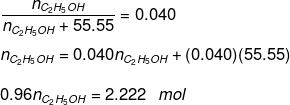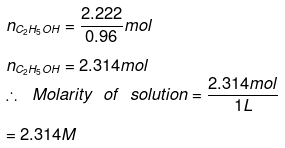Question 1.30: What will be the mass of one 12C atom in g?

1 mole of carbon atoms = 6.023 × 1023 atoms of carbon
= 12 g of carbon
Mass of one 12C atom = 12 g/6.022 × 1023
= 1.993 × 10–23 g.

Question 1.31: How many significant figures should be present in the of the following calculations?
(a)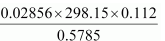(b) 5 × 5.364
(c) 0.0125 + 0.7864 + 0.0215

(a)Least precise number of calculation = 0.112
Number of significant figures in the Ans = Number of significant figures in the least precise number = 3

(b) 5 × 5.364
Least precise number of calculation = 5.364
Number of significant figures in the Ans = Number of significant figures in 5.364 = 4

(c) 0.0125 + 0.7864 + 0.0215
Since the least number of decimal places in each term is four, the number of significant figures in the Ans: is also 4.

Question 1.32: Use the data given in the following table to calculate the molar mass of naturally occurring argon isotopes: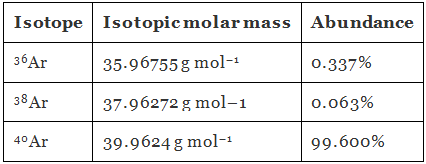Molar mass of argon: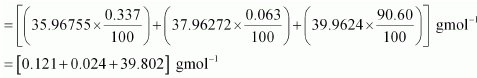= 39.947 g mol–1

Question 1.33: Calculate the number of atoms in each of the following:
(a) 52 moles of Ar
(b) 52 u of He
(c) 52 g of He

(a) 1 mole of Ar = 6.022 × 1023 atoms of Ar
52 mol of Ar = 52 × 6.022 × 1023 atoms of Ar
= 3.131 × 1025 atoms of Ar

(b) 1 atom of He = 4 u of He
Or,
4 u of He = 1 atom of He
1 u of He= 1/4 atom of He
52 u of He = 52 /4 atoms of He
= 13 atoms of He

(c) 4 g of He = 6.022 × 1023 atoms of He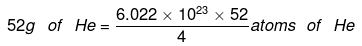= 7.8286 × 1024 atoms of He.

Question 1.34: A welding fuel gas contains carbon and hydrogen only. Burning a small sample of it in oxygen gives 3.38 g carbon dioxide, 0.690 g of water and no other products. A volume of 10.0 L (measured at STP) of this welding gas is found to weigh 11.6 g.
Calculate:
(a) Empirical formula
(b) Molar mass of the gas
(c) Molecular formula

a) 1 mole (44 g) of CO2 contains 12 g of carbon.
3.38 g of CO2 will contain carbon = 12 g/44 g × 3.38 g = 0.9217 g
18 g of water contains 2 g of hydrogen. = 2 g/18 g × 0.690
0.690 g of water will contain hydrogen = 0.0767 g
Since carbon and hydrogen are the only constituents of the compound, the total mass of the compound is:
= 0.9217 g + 0.0767 g
= 0.9984 g
Percent of C in the compound = 0.9217 g/0.9984 × 100
= 92.32%
Percent of H in the compound = 0.0767 g/0.9984 × 100
= 7.68%
Moles of carbon in the compound = 92.32/12.00
= 7.69
Moles of hydrogen in the compound = 7.68
∴ Ratio of carbon to hydrogen in the compound = 7.69 : 7.68
= 1: 1
Hence, the empirical formula of the gas is CH.

(b) Given,
Weight of 10.0 L of the gas (at S.T.P) = 11.6 g
∴ Weight of 22.4 L of gas at STP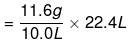= 25.984 g≈ 26 g.

Hence, the molar mass of the gas is 26 g.

(c) Empirical formula mass of CH = 12 + 1 = 13 g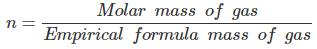= 26 g/13 gn = 2
Molecular formula of gas = (CH) = C2H2

Question 1.35: Calcium carbonate reacts with aqueous HCl to give CaCl2 and CO2 according to the reaction,
CaCO3(s) + 2HCl(aq) → CaCl2(aq) + CO2(g) + H2O(l)
What mass of CaCO3 is required to react completely with 25 mL of 0.75 M HCl?

0.75 M of HCl

≡ 0.75 mol of HCl are present in 1 L of water
≡ [(0.75 mol) × (36.5 g mol–1)] HCl is present in 1 L of water
≡ 27.375 g of HCl is present in 1 L of water

Thus, 1000 mL of solution contains 27.375 g of HCl.
Amount of HCl present in 25 mL of solution

= 27.375 g/1000 mL × 25 mL
= 0.6844 g

From the given chemical equation,
CaCO3(g) + 2HCl(aq) → CaCl2(aq) + CO2(g) + H2O(l)
2 mol of HCl (2 × 36.5 = 73 g) react with 1 mol of CaCO3 (100 g).
∴ Amount of CaCO3 that will react with 0.6844 g = (100/73) × 0.6844
= 0.9375 g

Question 1.36: Chlorine is prepared in the laboratory by treating manganese dioxide (MnO2) with aqueous hydrochloric acid according to the reaction
4 HCl(aq) + MnO2(s) → 2 H2O(l) + MnCl2(aq) + Cl2(g)
How many grams of HCl react with 5.0 g of manganese dioxide?

1 mol [55 + 2 × 16 = 87 g] MnO2 reacts completely with 4 mol [4 × 36.5 = 146 g] of HCl.
5.0 g of MnO2 will react with

= 146 g/87 g × 5.0 g of HCl
= 8.4 g of HCl

Hence, 8.4 g of HCl will react completely with 5.0 g of manganese dioxide.

The document NCERT Solutions - Basic Concepts of Chemistry (Part - 1) Class 11 Notes | EduRev is a part of the Class 11 Course NCERTs for NEET.
All you need of Class 11 at this link: Class 11Use Code STAYHOME200 and get INR 200 additional OFF Use Coupon Code
All Tests, Videos & Notes of Class 11: Class 11

### Up next## NCERTs for NEET

212 docs

### Up nextTrack your progress, build streaks, highlight & save important lessons and more!

,

,

,

,

,

,

,

,

,

,

,

,

,

,

,

,

,

,

,

,

,

;